• 多视图几何（Three-dimensional computer vision: a geometric viewpoint）（网页复制）
• 计算机视觉巨著《计算机视觉中的多视图几何(中文版) 》高清带书签
• 多视图几何1.前言1.1 多视图几何概念2. 基本原理2.1 对极几何2.2 基础矩阵2.2.1 基础矩阵推导2.2.2 求解基础矩阵3. 实验过程3.1 实验数据准备3.2 实验代码3.3 实验结果及分析4. 实验中遇到的问题 1.前言 1.1 多视图...
多视图几何1.前言1.1 多视图几何概念2. 基本原理2.1 对极几何2.2  基础矩阵2.2.1 基础矩阵推导2.2.2 求解基础矩阵3. 实验过程3.1 实验数据准备3.2 基础矩阵的计算3.2.1 实验代码3.2.2 实验结果及分析3.3 极点与极线3.3.1 实验代码3.3.2 实验结果及分析4. 实验中遇到的问题
1.前言
1.1 多视图几何概念
多视图几何是利用在不同视点所拍摄图像间的关系，来研究照相机之间或者特征之间关系的一门科学。
多视图几何（Multiple View Geometry）多视图几何主要研究用几何的方法，通过若干幅二维图像，来恢复三维物体。换言之就是研究三维重构，主要应用与计算机视觉中。
三维重构，即如何从静止物体不同视点下的图像中恢复物体三维几何结构信息。
在三维重构的过程中摄像机标定是一个极其重要环节，摄像机标定的研究分为三个部分：
（1）基于场景结构信息的标定；
（2）基于摄像机主动信息（纯旋转）的自标定；
（3）不依赖场景结构和摄像机主动信息的自标定。
2. 基本原理
2.1 对极几何
对极几何（Epipolar Geometry）描述的是两幅视图之间的内在射影关系，与外部场景无关，只依赖于摄像机内参数和这两幅试图之间的的相对姿态。
如下图所示，X为空间中的一点，x和x’为X在不同像平面中的投影，其中X与C, C’, x, x’ 共面。
tips：如果知道空间中点X在一幅图像中的投影点x，是无法确定空间中点X的位置以及X在其他图像中的投影点。
这里可以明确如下概念：

对极平面束（epipolar pencil）：以基线为轴的平面束；下图给出了包含两个平面的对极平面束。

对极平面（epipolar plane）：任何包含基线的平面都称为对极平面，或者说是对极平面束中的平面；例如，下图中的平面π就是一个对极平面。

对极点（epipole）：摄像机的基线与每幅图像的交点；即下图中的点e和e’。

对极线（epipolar line）：对极平面与图像的交线；例如，上图中的直线l和l’。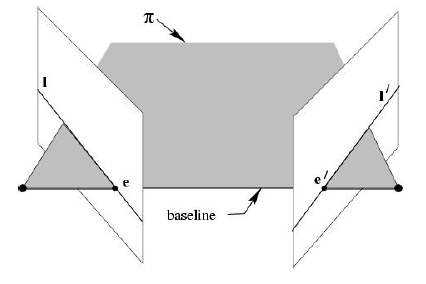点x、x’与摄像机中心C和C’是共面的，并且与空间点X也是共面的，这5个点共面于平面π，这是一个最本质的约束，即5个点决定了一个平面π。
由该约束，可以推导出一个重要性质：由图像点x和x’反投影的射线共面，并且，在平面π上。 在搜索点对应中，该性质非常重要
由此引出基础矩阵的概念。
2.2  基础矩阵
2.2.1 基础矩阵推导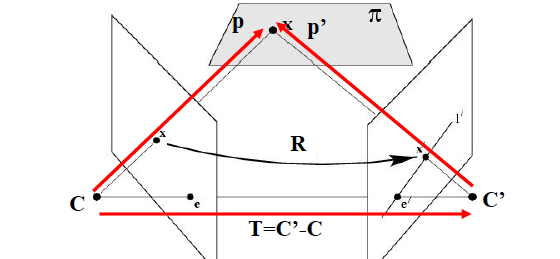如上图所示，设X分别以C,C’为坐标原点，x的坐标被表示为p和p’，则有
$p=Rp'+T$
其中R、T表示在两个坐标系之间的旋转和位移。
在以C为坐标系原点的时候有：$x=Kp \Rightarrow p=K^{-1}x$
在以C’为坐标系原点的时候有：$x'=K'p' \Rightarrow p'=K'^{-1}x'$
根据三点共面的性质可以知道：
$(p-T)^T(T×p)=0$
又因为$p=Rp'+T$得到$p-T=Rp'$，所以上式可化简如下：
$(R^Tp')^T(T×p)=0$
其中$T×p=Sp$，又$S=\begin{bmatrix} 0& -T_z & T_y\\ T_z& 0 &-T_x \\ -T_y& T_x & 0 \end{bmatrix}$
所以$(R^Tp')^T(T×p)=0$可写为下式：
$(R^Tp')^T(Sp)=0\Rightarrow (p'^TR)(Sp)=0\Rightarrow p'^TEp=0$
最终得到的如上式为本质矩阵。本质矩阵描述了空间中的点X在两个坐标系中的坐标对应关系。
根据上一篇博客中提到的$K$和$K'$分别为两个相机的内参矩阵，有：
$p=K^{-1}x \quad \quad p'=K'^{-1}x'$
用上式代替 $p'^TEp=0$ 中的p和p’ 可以得到下式：
$(K^{-1}x)^TE(K'^{-1}x')=0$
$\Rightarrow x'^TK^{-T}EK^{-1}x=0$
$\Rightarrow x'^TFx=0$
经推到得到了上述基础矩阵$F$,基础矩阵描述了空间中的点在两个像平面中的坐标对应关系。
注意：
分别以C,C’为坐标原点，x的坐标被表示为p和p’，则有下式：
$p'^TEp=0$
其实E表示p’和p之间的位置关系，E为本质矩阵。
2.2.2 求解基础矩阵
基本矩阵是由下述方程定义：
$x'^TFx=0$
其中$x↔x'x↔x′$是两幅图像的任意一对匹配点。由于每一组点的匹配提供了计算$F$系数的一个线性方程，当给定至少7个点（3×3的齐次矩阵减去一个尺度，以及一个秩为2的约束），方程就可以计算出未知的$F$。
记点的坐标为$x=(x,y,1)^T，x'=(x',y',1)^T，$则对应的方程为:
$\begin{bmatrix} x& x& 1 \end{bmatrix}\begin{bmatrix} f_{11}& f_{12}& f_{13}\\ f_{21}& f_{22}& f_{23}\\ f_{31}& f_{32}& f_{33}\\ \end{bmatrix}\begin{bmatrix} x'\\ y'\\ 1 \end{bmatrix}=0$
展开后有：
$x'xf_{11}+x'yf_{12}+x'f_{13}+y'xf_{21}+y'yf_{22}+y'f_{23}+xf_{31}+yf_{32}+f_{33}=0$
把矩阵$F$写成列向量的形式，则有：
$[x'x \quad x'y \quad x' \quad y'x \quad y'y \quad y' \quad x \quad y \quad 1]f=0$
给定$n$组点的集合，我们有如下方程：
$Af=\begin{bmatrix} x_1'x_1& x_1'y_1&x_1' & y_1'x_1& y_1'y_1& y_1' & x_1 & y_1& 1\\ ⋮ & ⋮& ⋮& ⋮& ⋮ &⋮ & ⋮& ⋮&⋮ \\ x_n'x_n &x_n'y_n &x_n' & y_n'x_n &y_n'y_n &y_n' &x_n &y_n &1 \end{bmatrix}f=0$
如果存在确定（非零）解，则系数矩阵$A$的秩最多是$8$。由于F是齐次矩阵，所以如果矩阵$A$的秩为$8$，则在差一个尺度因子的情况下解是唯一的。可以直接用线性算法解得。
如果由于点坐标存在噪声则矩阵$A$的秩可能大于$8$（也就是等于$9$，由于$A$是$n*9$的矩阵）。这时候就需要求最小二乘解，这里就可以用SVD来求解，ff的解就是系数矩阵$A$最小奇异值对应的奇异向量，也就是$A$奇异值分解后$A=UDV^T$
中矩阵$V$的最后一列矢量，这是在解矢量$f$在约束$\left \| f\right \|$下取$\left \|Af \right \|$最小的解。
求解基础矩阵可分为如下两步：

求线性解。由系数矩阵$A$最小奇异值对应的奇异矢量$f$求的$F$。
奇异性约束。是最小化Frobenius范数$\left \|F-F' \right \|$的F’代替F。

8点求解基础矩阵算法是计算基本矩阵的最简单的方法。为了提高解的稳定性和精度，往往会对输入点集的坐标先进行归一化处理。
3. 实验过程
3.1 实验数据准备
在本次实验中，拍摄三组不同的图像来求解基础矩阵并且观察极线的分布，数据如下：
第一组数据：左右拍摄，极点位于图像平面上（左右拍摄）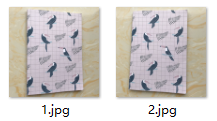第二组数据：像平面接近平行，极点位于无穷远（左右平移）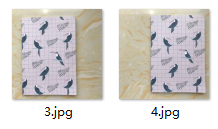第三组数据：图像拍摄位置位于前后（远近不同）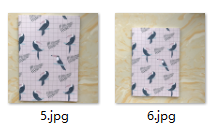3.2 基础矩阵的计算
关于基础矩阵的计算，本次实验设计7点、8点、10点计算基础矩阵，对于匹配点的个数对于基础矩阵的结果有何影响。
3.2.1 实验代码
from PIL import Image
from numpy import *
from pylab import *
import numpy as np
from PCV.geometry import camera
from PCV.geometry import homography
from PCV.geometry import sfm
from PCV.localdescriptors import sift
# Read features
# 载入图像，并计算特征
im1 = array(Image.open('data/5.jpg'))
sift.process_image('data/5.jpg', 'im5.sift')
l1, d1 = sift.read_features_from_file('im5.sift')
im2 = array(Image.open('data/6.jpg'))
sift.process_image('data/6.jpg', 'im6.sift')
l2, d2 = sift.read_features_from_file('im6.sift')
# 匹配特征
matches = sift.match_twosided(d1, d2)
ndx = matches.nonzero()
# 使用齐次坐标表示，并使用 inv(K) 归一化
x1 = homography.make_homog(l1[ndx, :2].T)
ndx2 = [int(matches[i]) for i in ndx]
x2 = homography.make_homog(l2[ndx2, :2].T)
x1n = x1.copy()
x2n = x2.copy()
print(len(ndx))
figure(figsize=(16,16))
sift.plot_matches(im1, im2, l1, l2, matches, True)
show()
# Don't use K1, and K2
#def F_from_ransac(x1, x2, model, maxiter=5000, match_threshold=1e-6):
def F_from_ransac(x1, x2, model, maxiter=5000, match_threshold=1e-6):
""" Robust estimation of a fundamental matrix F from point
correspondences using RANSAC (ransac.py from
http://www.scipy.org/Cookbook/RANSAC).
input: x1, x2 (3*n arrays) points in hom. coordinates. """
from PCV.tools import ransac
data = np.vstack((x1, x2))
d = 20 # 20 is the original
# compute F and return with inlier index
F, ransac_data = ransac.ransac(data.T, model,8, maxiter, match_threshold, d, return_all=True)
return F, ransac_data['inliers']
# find E through RANSAC
# 使用 RANSAC 方法估计 E
model = sfm.RansacModel()
F, inliers = F_from_ransac(x1n, x2n, model, maxiter=5000, match_threshold=1e-4)
print(len(x1n))
print(len(inliers))
# 计算照相机矩阵（P2 是 4 个解的列表）
P1 = array([[1, 0, 0, 0], [0, 1, 0, 0], [0, 0, 1, 0]])
P2 = sfm.compute_P_from_fundamental(F)
# triangulate inliers and remove points not in front of both cameras
X = sfm.triangulate(x1n[:, inliers], x2n[:, inliers], P1, P2)
# plot the projection of X
cam1 = camera.Camera(P1)
cam2 = camera.Camera(P2)
x1p = cam1.project(X)
x2p = cam2.project(X)
figure()
imshow(im1)
gray()
plot(x1p, x1p, 'o')
#plot(x1, x1, 'r.')
axis('off')
figure()
imshow(im2)
gray()
plot(x2p, x2p, 'o')
#plot(x2, x2, 'r.')
axis('off')
show()
figure(figsize=(16, 16))
im3 = sift.appendimages(im1, im2)
im3 = vstack((im3, im3))
imshow(im3)
cols1 = im1.shape
rows1 = im1.shape
for i in range(len(x1p)):
if (0<= x1p[i]<cols1) and (0<= x2p[i]<cols1) and (0<=x1p[i]<rows1) and (0<=x2p[i]<rows1):
plot([x1p[i], x2p[i]+cols1],[x1p[i], x2p[i]],'c')
axis('off')
show()
print(F)
x1e = []
x2e = []
ers = []
for i,m in enumerate(matches):
if m>0: #plot([locs1[i],locs2[m]+cols1],[locs1[i],locs2[m]],'c')
x1=int(l1[i])
y1=int(l1[i])
x2=int(l2[int(m)])
y2=int(l2[int(m)])
# p1 = array([l1[i], l1[i], 1])
# p2 = array([l2[m], l2[m], 1])
p1 = array([x1, y1, 1])
p2 = array([x2, y2, 1])
# Use Sampson distance as error
Fx1 = dot(F, p1)
Fx2 = dot(F, p2)
denom = Fx1**2 + Fx1**2 + Fx2**2 + Fx2**2
e = (dot(p1.T, dot(F, p2)))**2 / denom
x1e.append([p1, p1])
x2e.append([p2, p2])
ers.append(e)
x1e = array(x1e)
x2e = array(x2e)
ers = array(ers)
indices = np.argsort(ers)
x1s = x1e[indices]
x2s = x2e[indices]
ers = ers[indices]
x1s = x1s[:20]
x2s = x2s[:20]
figure(figsize=(16, 16))
im3 = sift.appendimages(im1, im2)
im3 = vstack((im3, im3))
imshow(im3)
cols1 = im1.shape
rows1 = im1.shape
for i in range(len(x1s)):
if (0<= x1s[i]<cols1) and (0<= x2s[i]<cols1) and (0<=x1s[i]<rows1) and (0<=x2s[i]<rows1):
plot([x1s[i], x2s[i]+cols1],[x1s[i], x2s[i]],'c')
axis('off')
show()

3.2.2 实验结果及分析
① 第一组数据
七点匹配点计算基础矩阵：
控制台输出：
461
461
36
[[  1.97239734e-07  -4.26561273e-05   2.52904527e-02]
[  4.24989402e-05  -1.90178422e-06  -2.44330900e-02]
[ -2.44701559e-02   2.30334778e-02   1.00000000e+00]]

结果图：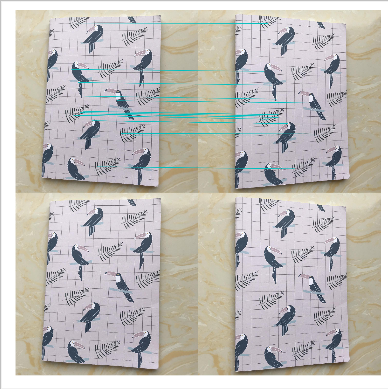八点匹配点计算基础矩阵：
控制台输出：
461
461
29
[[ -2.55224960e-07  -3.00786795e-07   1.58976498e-03]
[  2.89707522e-06   1.45441317e-07  -1.46080960e-02]
[ -2.81378730e-03   1.23999326e-02   1.00000000e+00]]

结果图：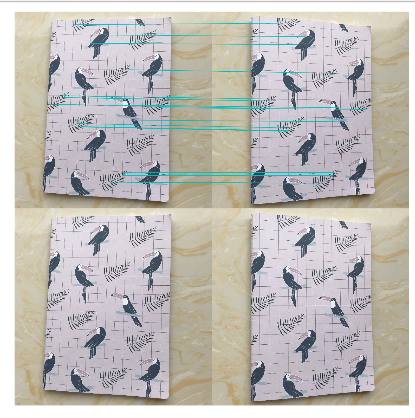十点匹配点计算基础矩阵：
控制台输出：
processed tmp.pgm to im1.sift
processed tmp.pgm to im2.sift
461
461
36
[[  6.79062229e-06  -3.58141837e-06  -3.86536085e-02]
[  4.48539039e-06  -1.53238078e-07  -5.44511839e-03]
[  2.94374668e-02   3.04050052e-03   1.00000000e+00]]

结果图：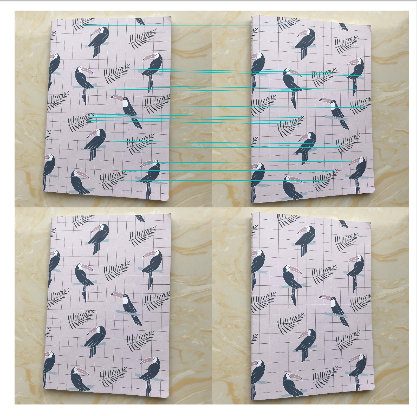② 第二组数据
七点匹配点计算基础矩阵：
控制台输出：
734
734
32
[[  4.41348662e-08  -3.42650050e-06   2.34914137e-03]
[  3.37627195e-06   3.86920082e-08  -2.77634118e-03]
[ -2.51280140e-03   1.50309243e-03   1.00000000e+00]]

结果图：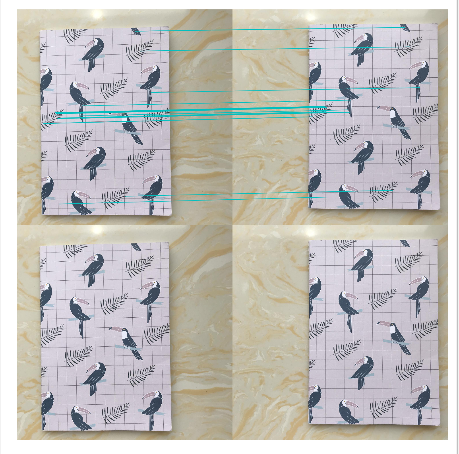八点匹配点计算基础矩阵：
控制台输出：
734
734
59
[[  5.13681399e-08  -2.72006999e-06   2.33030990e-03]
[  2.71521610e-06   1.85826640e-08  -2.25616582e-03]
[ -2.51198132e-03   1.22928879e-03   1.00000000e+00]]

结果图：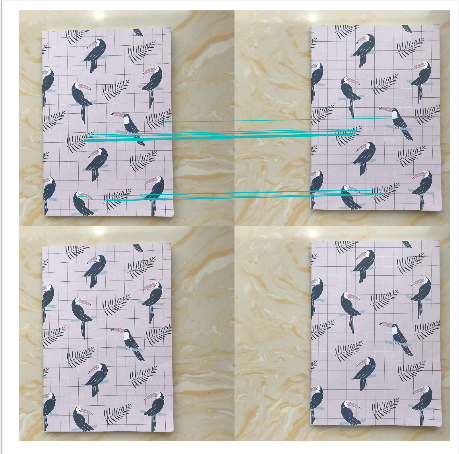十点匹配点计算基础矩阵：
控制台输出：
734
734
46
[[  3.32345027e-08  -5.21542621e-06   1.99979624e-03]
[  5.14872251e-06   2.89078827e-08  -6.53046230e-03]
[ -2.23058142e-03   4.65965814e-03   1.00000000e+00]]

结果图：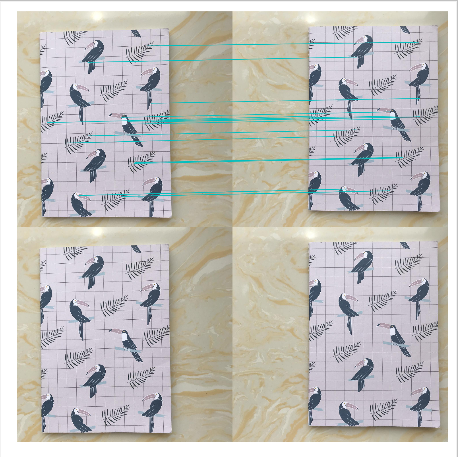③第三组数据
七点匹配点计算基础矩阵：
控制台输出：
632
632
28
[[  2.87480615e-06  -2.51191641e-04   1.83003566e-01]
[  2.49457112e-04   3.69779415e-06  -1.27817047e-01]
[ -1.69252496e-01   1.14233511e-01   1.00000000e+00]]

结果图：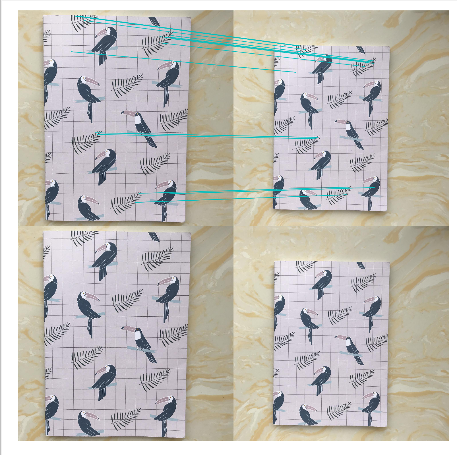八点匹配点计算基础矩阵：
控制台输出：
632
632
32
[[  3.78497574e-08   2.12332184e-05  -3.28211216e-02]
[ -2.20359610e-05   4.36071279e-07   2.63780580e-02]
[  3.62485855e-02  -2.94763915e-02   1.00000000e+00]]

结果图：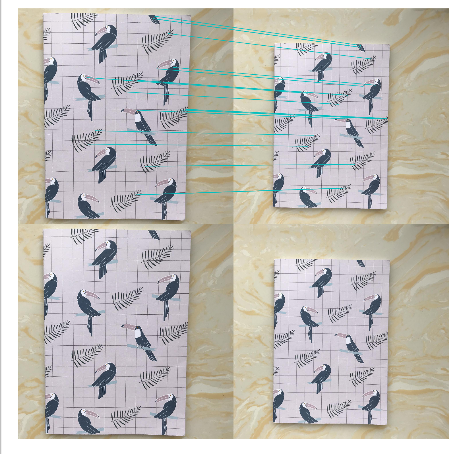十点匹配点计算基础矩阵：
控制台输出：
632
632
53
[[  4.66996990e-08  -2.04474321e-05   1.99506699e-02]
[  2.07208420e-05   2.14957900e-07  -1.06213988e-02]
[ -2.00422549e-02   9.28538206e-03   1.00000000e+00]]

结果图：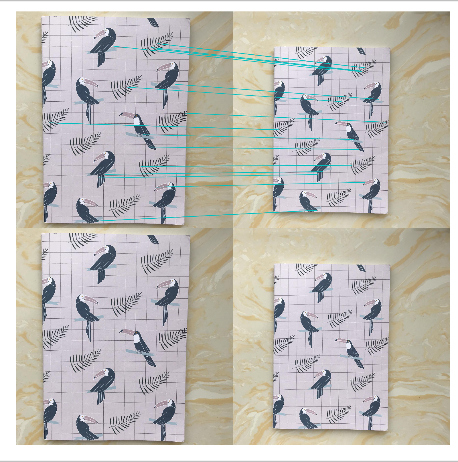实验分析：
（1）由第一组的实验结果分析可得：随着改变参数7、8、10，即匹配点的个数，得到的基础矩阵是各不相同的，对基础矩阵的有非常大的影响；除此之外匹配点的个数对于最终得到的特征匹配图也有较大的影响，其规律不是特别明显，但当参数较大时，匹配线的数量较多。
（2）远近不同的图片组经过算法匹配效果相对于远近相同的图片组效果要差一些，甚至出现了明显的错误匹配，第一组的图片匹配点较多，但是优化后所剩下的较少，第二张匹配点相对第一组较少，但是优化过后明显特征的匹配点仍被保留。
（3）sift算法本身有时候会因为匹配点附近太过相似而出现错误匹配，这个算法便能在这个基础上相对优化，避开错误的匹配，但是以上的结果都出现了一个情况，优化后的结果仍存在错误的匹配点，整体效果并不好，由于图像远近以及光线的影响，呈现的效果也不够理想，而相对的，远近相同的测试图片效果会比远近相差较大的图片要好，更容易匹配。
（4）在本次实验中，三组数据相较来说，平移变换图像相比较其他两种构图方式的图像有着较多的特征匹配数，因为平移变换对物体特征点的影响较小，而远近和左右角度变换都会在不同程度上影响到特征点的收集和遮蔽。
3.3 极点与极线
3.3.1 实验代码
import numpy as np
import cv2 as cv
from matplotlib import pyplot as plt
def drawlines(img1, img2, lines, pts1, pts2):
''' img1 - image on which we draw the epilines for the points in img2
lines - corresponding epilines '''
r, c = img1.shape
img1 = cv.cvtColor(img1, cv.COLOR_GRAY2BGR)
img2 = cv.cvtColor(img2, cv.COLOR_GRAY2BGR)
for r, pt1, pt2 in zip(lines, pts1, pts2):
color = tuple(np.random.randint(0, 255, 3).tolist())
x0, y0 = map(int, [0, -r / r])
x1, y1 = map(int, [c, -(r + r * c) / r])
img1 = cv.line(img1, (x0, y0), (x1, y1), color, 1)
img1 = cv.circle(img1, tuple(pt1), 5, color, -1)
img2 = cv.circle(img2, tuple(pt2), 5, color, -1)
return img1, img2
img1 = cv.imread('data/1.jpg', 0)  # queryimage # left image
img2 = cv.imread('data/2.jpg', 0)  # trainimage # right image
sift = cv.xfeatures2d.SIFT_create()
# find the keypoints and descriptors with SIFT
kp1, des1 = sift.detectAndCompute(img1, None)
kp2, des2 = sift.detectAndCompute(img2, None)
# FLANN parameters
FLANN_INDEX_KDTREE = 1
index_params = dict(algorithm=FLANN_INDEX_KDTREE, trees=5)
search_params = dict(checks=50)
flann = cv.FlannBasedMatcher(index_params, search_params)
matches = flann.knnMatch(des1, des2, k=2)
good = []
pts1 = []
pts2 = []
# ratio test as per Lowe's paper
for i, (m, n) in enumerate(matches):
if m.distance < 0.8 * n.distance:
good.append(m)
pts2.append(kp2[m.trainIdx].pt)
pts1.append(kp1[m.queryIdx].pt)
pts1 = np.int32(pts1)
pts2 = np.int32(pts2)
F, mask = cv.findFundamentalMat(pts1, pts2, cv.FM_LMEDS)
# We select only inlier points
pts1 = pts1[mask.ravel() == 1]
pts2 = pts2[mask.ravel() == 1]
# Find epilines corresponding to points in right image (second image) and
# drawing its lines on left image
lines1 = cv.computeCorrespondEpilines(pts2.reshape(-1, 1, 2), 2, F)
lines1 = lines1.reshape(-1, 3)
img5, img6 = drawlines(img1, img2, lines1, pts1, pts2)
# Find epilines corresponding to points in left image (first image) and
# drawing its lines on right image
lines2 = cv.computeCorrespondEpilines(pts1.reshape(-1, 1, 2), 1, F)
lines2 = lines2.reshape(-1, 3)
img3, img4 = drawlines(img2, img1, lines2, pts2, pts1)
plt.subplot(121), plt.imshow(img5)
plt.subplot(122), plt.imshow(img3)
plt.show()

3.3.2 实验结果及分析
① 第一组数据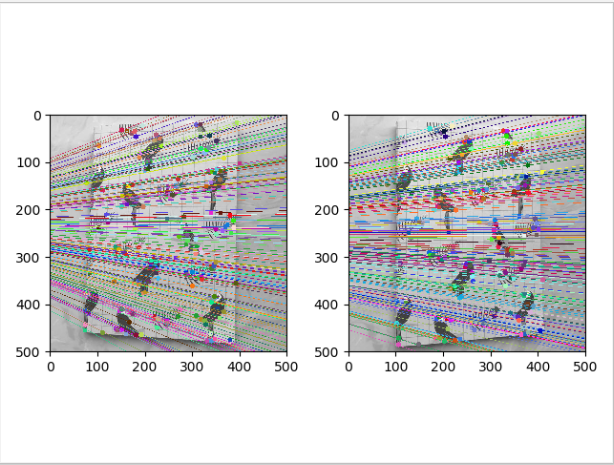② 第二组数据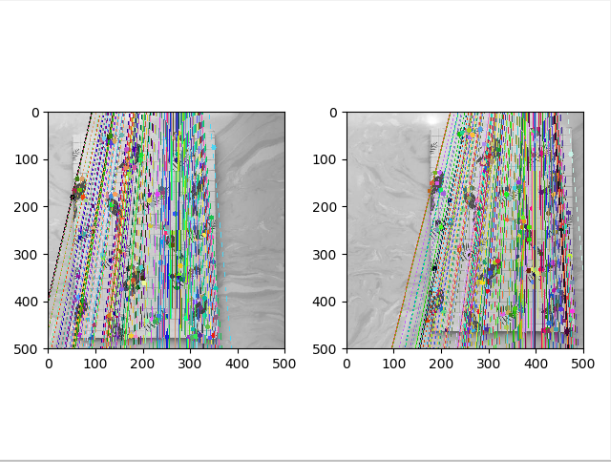③ 第三组数据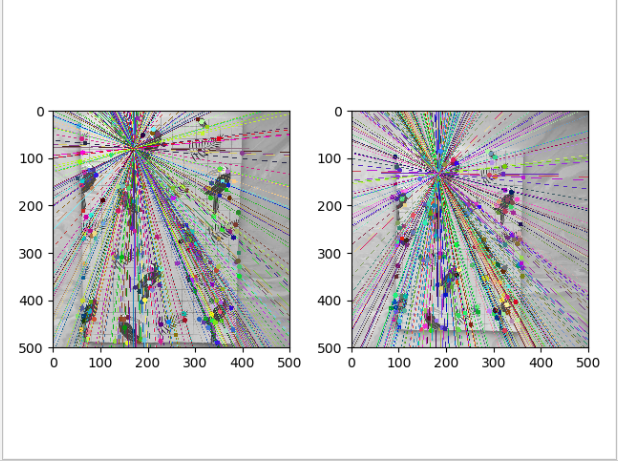实验分析：

对于第三组实验数据来说，极点为途中所有直线的交点处，由于改组图像为前后远近不同的图像组，所以该图像组的极线呈散射状，极点位于所有极线的交点处。

4. 实验中遇到的问题
在本次实验中遇到如下问题，在代码运行过程中遇到如下报错：
ImportError: cannot import name 'ransac'

解决方法：
将代码中的from PCV.geometry import ransac改为from PCV.tools import ransac即可，因为ransac.py文件夹在PCV下的tools文件夹内，不在geometry 内。


展开全文• 计算机视觉中的多视图几何(中文版)。计算机视觉中的多视图几何(中文版)计算机视觉中
• ## 计算机视觉中的多视图几何

千次下载 热门讨论 2011-12-03 09:40:01
计算机视觉中的多视图几何，这是第一版的中文版，目前还没有第二版的中文版，但是第二版更新不是太多，英文阅读不流利的可以先看此版，再去查阅英文版的更新，效率比较高，文档比较大只好分开传。
• 计算机视觉中的多视图几何，完整清晰版，安徽大学韦穗
• 计算机视觉中的多视图几何（中文版）200-484，计算机视觉中的多视图几何（中文版）200-484，计算机视觉中的多视图几何（中文版）200-484计算机视觉
• 文章目录多视图几何1 外极几何1.1 一个简单的数据集1.2 用Matplotlib绘制三维数据1.3 计算F：八点法1.4 外极点和外极线2 照相机和三维结构的计算2.1 三角剖分2.2 由三维点计算照相机矩阵2.3 由基础矩阵计算照相机...
文章目录多视图几何1 外极几何1.1 一个简单的数据集1.2 用Matplotlib绘制三维数据1.3 计算F：八点法1.4 外极点和外极线2 照相机和三维结构的计算2.1 三角剖分2.2 由三维点计算照相机矩阵2.3 由基础矩阵计算照相机矩阵2.3.1 投影重建2.3.1 度量重建3 多视图重建3.1 稳健估计基础矩阵3.2 三维重建示例4 立体图像
多视图几何
多视图几何是利用在不同视点所拍摄的图像间的关系，来研究照相机之间或者特征之间关系的一门学科。图像的特征通常就是兴趣点。
1 外极几何
如果一个场景的两个视图以及视图中的对应图像点，那么根据照相机间的空间相对位置关系、照相机的性质以及三维场景点的位置，可以得到对这些图像点的几何约束关系，即外极几何。
1.1 一个简单的数据集
使用下面的程序加载两个图像、三个视图中的所有图像特征点、对应不同视图图像点重建后的三维点以及照相机参数矩阵。
import my_camera

im1 = array(Image.open('001.jpg'))
im1 = array(Image.open('002.jpg'))

points2D = [loadtxt('2D/00' + str(i + 1) + '.corners').T for i in range(3)]

points3D = loadtxt('3D/p3d').T

corr = genfromtxt('2D/nview-corners', dtype = 'int', missing = '*')

P = [my_camera.Camera(loadtxt('2D/00' + str(i + 1) + '.P')) for i in range(3)]

使用loadtxt()函数读取文本文件到NumPy数组中，因为并不是所有的点都可见，或都能够成功匹配到所有的视图，所以对应数据里包含了缺失的数据。genfromtxt()函数通过将缺失的数值填充为-1来解决这个问题。
将上述代码保存到load_vggdata.py，然后使用命令excefile()可以运行该脚本，从而获取数据：
execfile('load_vggdata.py')

结果报错：NameError: name ‘execfile’ is not defined
这是由于execfile()在python3中已被废除，可以使用代替函数： exec(open(filename).read())来完成。
下面来可视化这些数据，将三维的点投影到一个视图上，然后和观测到的图像点比较：
X = vstack((points3D, ones(points3D.shape)))
x = P.project(X)

figure()
imshow(im1)
plot(points2D, points2D, '*')
axis('off')

figure()
imshow(im1)
plot(x, x, 'r.')
axis('off')

show()图1 图像点与三维投影点
上边的代码绘制出第一个视图以及该视图中的图像点。仔细观察发现第二幅图比第一幅图多一些点，这些多出来的点是从视图2和视图3重建出来的，而不是在视图1中。
1.2 用Matplotlib绘制三维数据
为了可视化三维重建结果，需要绘制出三维图像。Matplotlib中的mplot3d工具包可以方便地绘制出三维点、线、等轮廓线、表面以及其他基本图形组件，还可以通过图像窗口控件实现三维旋转和缩放。
可以通过在axes对象中加上projection=“3d”关键字实现三维绘图，如下：
from mpl_toolkits.mplot3d import axes3d

fig = figure()
ax = fig.gca(projection = "3d")

X, Y, Z = axes3d.get_test_data(0.25)

ax.plot(X.flatten(), Y.flatten(), Z.flatten(), 'o')

show()

get_test_data()函数在x，y空间按照设定的空间间隔参数来产生均匀的采样点。压平这些网格会产生三列数据点，然后可以将其输入plot()函数，就可以在立体表面画出这些三维点。图2 立体表面的三维点
通过画出Merton样本数据来观察三维点的效果：
fig = figure()
ax = fig.gca(projection = "3d")
ax.plot(points3D, points3D, points3D, 'k.')

show()图3 建筑墙体和屋顶上的三维点
1.3 计算F：八点法
八点法是通过对应点来计算基础矩阵的算法。
下面的代码写入八点法中最小化||Af||的函数：
def compute_fundamental(x1, x2):
n = x1.shape

if x2.shape != n:
raise ValueError("Number of points don't match.")

A = zeros((n, 9))

for i in range(n):
A[i] = [x1[0, i] * x2[0, i], x1[0, i] * x2[1, i], x1[0, i] * x2[2, i],
x1[1, i] * x2[0, i], x1[1, i] * x2[1, i], x1[1, i] * x2[2, i],
x1[2, i] * x2[0, i], x1[2, i] * x2[q, i], x1[2, i] * x2[0, i]]

U, S, V = linalg.svd(A)
F = V[-1].reshape(3, 3)

U, S, V = linalg.svd(F)
S = 0
F = dot(U, dot(diag(S), V))

return F

通常使用SVD算法来计算最小二乘解。由于上面算法得出的解可能秩不为2，所以通过将最后一个奇异值置为0来得到秩最接近2的基础矩阵。
1.4 外极点和外极线
如果要获得一幅图像的外极点，只需要将F转置后输入下述函数：
def compute_epipole(F):
U, S, V = linalg.svd(F)
e = V[-1]

return e / e

def plot_epipolar_line(im, F, x, epipole = None, show_epipole = True):
m, n = im.shape[:2]
line = dot(F, x)

t = linspace(0, n, 100)
lt = array([(line + line * tt) / (-line) for tt in t])

ndx = (lt >= 0) & (lt < m)
plot(t[ndx], lt[ndx], linewidth = 2)

if show_epipole:
if epipole is None:
epipole = compute_epipole(F)
plot(epipole / epipole, epipole / epipole, 'r*')

上面的函数将x轴的范围作为直线的参数，因此直线超出图像边界的部分会被截断。如果show_epipole为真，外极点也会被画出来（如果输入参数没有外极点，则外极点会在程序中计算出来）。
可以在之前样本数据集的前两个视图上运行这两个函数：
ndx = (corr[:, 0] >= 0) & (corr[:, 1] >= 0)

x1 = points2D[:, corr[ndx, 0]]
x1 = vstack((x1, ones(x1.shape)))

x2 = points2D[:, corr[ndx, 1]]
x2 = vstack((x2, ones(x2.shape)))

F = sfm.compute_fundamental(x1, x2)

e = sfm.compute_epipole(F)

figure()
imshow(im1)

for i in range(5):
sfm.plot_epipolar_line(im1, F, x2[:, i], e, False)
axis('off')

figure()
imshow(im2)

for i in range(5):
plot(x2[0, i], x2[1, i], 'o')
axis('off')

show()

首先选择两幅图像的对应点，然后将他们转换为齐次坐标。然后画出了对应匹配点。在下图中用不同颜色将点和相应的外极线对应起来。图4 数据视图中的5个外极点
2 照相机和三维结构的计算
2.1 三角剖分
给定照相机参数模型，图像点可以通过三角剖分来恢复出这些点的三维位置。基本算法思想如下：
对于两个照相机P1，P2的视图，三维实物点X的投影点x1和x2，照相机方程定义了下列关系：由于图像噪声、照相机参数误差和其他系统误差，上面的方程可能没有精确解。需要用到SVD算法来得到三维点的最小二乘估值。
下面的函数用于计算一个点对的最小二乘三角剖分：
def triangulate_points(x1, x2, P1, P2)：
M = zeros((6, 6))
M[:3, :4] = P1
M[3:, :4] = P2
M[:3, 4] = -x1
M[3:, 5] = -x2

U, S, V = linalg.svd(M)
X = V[-1, :4]

return X / X

最后一个特征向量的前4个值就是齐次坐标系下的对应三维坐标，可以增加下面的函数来实现多个点的三角剖分：
def triangulate(x1, x2, P1, P2):
n = x1.shape
if x2.shape != n:
raise ValueError("Number of points don't match.")

X = [triangulate_points(x1[:, i], x2[:, i], P1, P2) for i in range(n)]

return array(X).T

这个函数的输入是两个图像点数组，输出为一个三维坐标数组。
可以利用下面的代码来实现三角剖分：
ndx = (corr[:, 0] >= 0) & (corr[:, 1] >= 0)

x1 = points2D[:, corr[ndx, 0]]
x1 = vstack((x1, ones(x1.shape)))
x2 = points2D[:, corr[ndx, 1]]
x2 = vstack((x2, ones(x2.shape)))

Xtrue = points3D[:, ndx]
Xtrue = vstack((Xtrue, ones(Xtrue.shape)))

Xest = sfm.triangulate(x1, x2, P.P, P,P)
print(Xest[:, :3])
print(Xtrue[:, :3])

from mpl_toolkits.mplot3d import axes3d

fig = figure()
ax = fig.gca(projection = '3d')
ax.plot(Xest, Xest, Xest, 'ko')
ax.plot(Xtrue, Xtrue, Xtrue, 'r.')

axis('equal')

show()

上面的代码首先利用前两个视图的信息来对三维点进行三角剖分，然后把前三个图像点的齐次坐标输出到控制台，最后绘制出灰度的最接近三维图像点。输出到控制台的信息如下：
[[ 1.03743725  1.56125273  1.40720017]
[-0.57574987 -0.55504127 -0.46523952]
[ 3.44173797  3.44249282  7.53176488]
[ 1.          1.          1.        ]]
[[ 1.0378863   1.5606923   1.4071907 ]
[-0.54627892 -0.5211711  -0.46371818]
[ 3.4601538   3.4636809   7.5323397 ]
[ 1.          1.          1.        ]]
算法估计出的三维图像点和实际图像点很接近。图5 使用照相机矩阵和点的对应关系三角剖分数据点
2.2 由三维点计算照相机矩阵
如果已经知道了一些三维点及其图像投影，可以使用直接线性变换的方法来计算照相机矩阵P。本质上这也是三角剖分方法的逆问题，也称照相机反切法。
相应代码如下：
def compute_P(x, X):
n = x.shape
if X.shape != n:
raise ValueError("Number of points don't match.")

M = zeros((3 * n, 12 + n))
for i in range(n):
M[3 * i, 0: 4] = X[:, i]
M[3 * i + 1, 4: 8] = X[:, i]
M[3 * i + 2, 8: 12] = X[:, i]
M[3 * i: 3 * i + 3, i + 12] = -x[:, i]

U, S, V = linalg.svd(M)

return V[-1, :12].reshape((3, 4))

该函数的输入参数为图像点和三维点，构造出上述所示的M矩阵。最后一个特征向量的前12个元素是照相机矩阵的元素，经过重新排列成矩阵形状后返回。
下面，在样本数据集上测试算法的性能。
corr = corr[:, 0]
ndx3D = where(corr >= 0)
ndx2D = corr[ndx3D]

x = points2D[:, ndx2D]
x = vstack((x, ones(x.shape)))
X = points3D[:, ndx3D]
X = vstack((X, ones(X.shape)))

Pest = my_camera.Camera(sfm.compute_P(x, X))

print(Pest.P / Pest.P[2, 3])
print(P.P / P.P[2, 3])

xest = Pest.project(X)

figure()
imshow(im1)
plot(x, x, 'bo')
plot(xest, xest, 'r.')
axis('off')

show()

上面的代码选出第一个视图中的一些可见点，将它们转换为齐次坐标表示，然后估计照相机矩阵。为了检查照相机矩阵的正确性，将他们归一化的格式（除以最有一个元素）打印到控制台。输出如下所示：
[[ 1.06520794e+00 -5.23431275e+01  2.06902749e+01  5.08729305e+02]
[-5.05773115e+01 -1.33243276e+01 -1.47388537e+01  4.79178838e+02]
[ 3.05121915e-03 -3.19264684e-02 -3.43703738e-02  1.00000000e+00]]
[[ 1.06774679e+00 -5.23448212e+01  2.06926980e+01  5.08764487e+02]
[-5.05834364e+01 -1.33201976e+01 -1.47406641e+01  4.79228998e+02]
[ 3.06792659e-03 -3.19008054e-02 -3.43665129e-02  1.00000000e+00]]图6 使用估计出的照相机矩阵计算视图1中的投影点
可以看出估计出的照相机矩阵和数据集计算出的照相机矩阵，它们的元素几乎完全相同。最后使用估计出的照相机矩阵投影这些三维点，然后绘制出投影后的结果，如上图所示，真实点用圆圈表示，估计出的照相机投影点用点表示。
2.3 由基础矩阵计算照相机矩阵
在两个视图场景中，照相机矩阵可以由基础矩阵恢复出来。研究分为两类：未标定的情况和已标定的情况。
2.3.1 投影重建
在没有任何照相机内参数知识的情况下，照相机矩阵只能通过射影变换恢复出来。也就是说，如果利用照相机信息来重建三维点，那么该重建只能由射影变换计算出来。（在这里不考虑角度和距离）
具体代码如下：
def skew(a):
return array([[0, -a, a], [a, 0, -a], [-a, a, 0]])

def compute_P_from_fundamental(F):
e = compute_epipole(F.T)
Te = skew(e)

return vstack((dot(Te, F.T).T, e)).T

2.3.1 度量重建
在已标定的情况下，重建会保持欧式空间中的一些度量特性（除了全局的尺度参数）。从本质矩阵恢复出的照相机矩阵中存在度量关系，但有四个可能解。因为只有一个解产生位于两个照相机前的场景，所以可以轻松选出。
具体代码如下：
def compute_P_from_essential(E):
U, S, V = svd(E)

if det(dot(U, V)) < 0:
V = -V

E = dot(U, dot(diag([1, 1, 0]), V))

Z = skew([0, 0, -1])
W = array([[0, -1, 0], [1, 0, 0], [0, 0, 1]])

P2 = [vstack((dot(U, dot(W, V)).T, U[:, 2])).T,
vstack((dot(U, dot(W, V)).T, -U[:, 2])).T,
vstack((dot(U, dot(W.T, V)).T, U[:, 2])).T,
vstack((dot(U, dot(W.T, V)).T, -U[:, 2])).T]

return P2

3 多视图重建
由于照相机的运动给我们提供了三维结构，所以这样计算三维重建的方法通常称为SfM。
假设照相机已经标定，计算重建可以分为下面4个步骤：

检测特征点，然后在两幅图之间匹配
由匹配计算基础矩阵
由基础矩阵计算照相机矩阵
三角剖分这些三维点

3.1 稳健估计基础矩阵
这部分类似于稳健计算单应性矩阵，当存在噪声和不正确的匹配时，需要估计基础矩阵。使用RANSAC方法并结合八点法来完成。
class RansacModel(object):
def __init__(self, debug = False):
self.debug = debug

def fit(self, data):
data = data.T
x1 = data[:3, :8]
x2 = data[3:, :8]

F = compute_fundamental_normalized(x1, x2)

return F

def get_error(self, data, F):
data = data.T
x1 = data[:3]
x2 = data[3:]

Fx1 = dot(F, x1)
Fx2 = dot(F, x2)
denom = Fx1 ** 2 + Fx ** 2 + Fx2 ** 2 + Fx2 ** 2

err = (diag(dot(x1.T, dot(F, x2)))) ** 2 / denom

return err

def compute_fundamental_normalized(x1, x2):
n = x1.shape
if x2.shape != n:
raise ValueError("Number of points don't match.")

x1 = x1 / x1
mean_1 = mean(x1[:2], axis = 1)
S1 = sqrt(2) / std(x1[:2])
T1 = array([[S1, 0, -S1 * mean_1], [0, S1, -S1 * mean_1], [0, 0, 1]])
x1 = dot(T1, x1)

x2 = x2 / x2
mean_2 = mean(x2[:2], axis = 1)
S2 = sqrt(2) / std(x2[:2])
T2 = array([[S2, 0, -S2 * mean_2], [0, S2, -S2 * mean_2], [0, 0, 1]])
x2 = dot(T2, x2)

F = compute_fundamental(x1, x2)

F = dot(T1.T, dot(F, T2))

return F / F[2, 2]

def F_from_ransac(x1, x2, model, maxiter = 5000, match_theshold = 1e-6):
import ransac

data = vstack((x1, x2))

F, ransac_data = ransac.ransac(data.T, model, 8, maxiter, match_theshold, 20, return_all = True)

return F, ransac_data['inliers']

这里返回最佳基础矩阵F，以及正确点的索引，可以知道那些匹配和F矩阵是一致的。与单应性矩阵估计相比，增加了默认的最大迭代次数，改变了匹配的阈值。
3.2 三维重建示例
在接下来的处理中，将该示例的处理代码分成若干块，使得代码更容易理解。首先，提取、匹配特征，然后估计基础矩阵和照相机矩阵：
K = array([[2394, 0, 932], [0, 2398, 628], [0, 0, 1]])

im1 = array(Image.open('alcatraz1.jpg'))
my_sift.process_image('alcatraz1.jpg', 'im1.sift')
l1, d1 = my_sift.read_features_from_file('im1.sift')

im2 = array(Image.open('alcatraz2.jpg'))
my_sift.process_image('alcatraz2.jpg', 'im2.sift')
l2, d2 = my_sift.read_features_from_file('im2.sift')

matches = my_sift.match_twosided(d1, d2)
ndx = matches.nonzero()

x1 = my_homography.make_homog(l1[ndx, :2].T)
ndx2 = [int(matches[i]) for i in ndx]
x2 = my_homography.make_homog(l2[ndx2, :2].T)

x1n = dot(inv(K), x1)
x2n = dot(inv(K), x2)

model = sfm.RansacModel()
E, inliers = sfm.F_from_ransac(x1n, x2n, model)

P1 = array([[1, 0, 0, 0], [0, 1, 0, 0], [0, 0, 0, 1]])
P2 = sfm.compute_P_from_essential(E)

在获得了标定矩阵后，对矩阵K进行硬编码。使用K的逆矩阵来对其进行归一化，并使用归一化的八点算法来运行Ransac估计，返回一个本质矩阵并保存正确匹配点的索引。从本质矩阵出发，可以计算出第二个照相机矩阵的四个可能解。
从照相机矩阵的列表中，挑选出经过三角剖分后，在两个照相机前郡含有场景点的照相机矩阵：
ind = 0
maxres = 0
for i in range(4):
X = sfm.triangulate(x1n[:, inliers], x2n[:, inliers], P1, P2[i])
d1 = dot(P1, X)
d2 = dot(P2[i], X)

if sum(d1 > 0) + sum(d2 > 0) > maxres:
maxres = sum(d1 > 0) + sum(d2 > 0)
ind = i
infront = (d1 > 0) & (d2 > 0)

X = sfm.triangulate(x1n[:, inliers], x2n[:, inliers], P1, P2[ind])
X = X[:, infront]

循环遍历这四个解，每次对对应于正确点的三维点进行三角剖分。将三角剖分后的图像投影回图像后，深度的符号由每个图像点的第三个数值给出。我们保存了正向最大深度的索引；对于和最优解一致的点，用相应的布尔变量保存了信息，这样可以取出真正在照相机前面的点。因为所有估计中都存在噪声和误差，所以即便使用正确的照相机矩阵，也存在一些点仍位于某个照相机后面的风险。首先获得正确的解，然后对这些正确的点进行三角剖分，最后保留位于照相机前方的点。
现在可以绘制出该三维重建：
cam1 = my_camera.Camera(P1)
cam2 = my_camera.Camera(P2)

x1p = cam1.project(X)
x2p = cam2.project(X)

x1p = dot(K, x1p)
x2p = dot(K, x2p)

figure()
imshow(im1)
gray()
plot(x1p, x1p, 'o')
plot(x1, x1, 'r.')
axis('off')

figure()
imshow(im2)
gray()
plot(x2p, x2p, 'o')
plot(x2, x2, 'r.')
axis('off')

show()

将这些三维点投影后，可以通过乘以标定矩阵的方式来弥补初始归一化对点的影响。
程序在运行中出现一个问题：图7 三维重建中遇到的问题
最后得到的结果如下图所示：图8 使用图像匹配从一对图像中计算三维重建
从图中可以看到，二次投影后的点和原始特征位置不完全匹配，但是相当接近。可以进一步调整照相机矩阵来提高重建和二次投影的性能。
4 立体图像
一个多视图成像的特殊例子是立体视觉，即使用两台只有水平偏移的照相机观测同一场景。当照相机的位置如上设置，两幅图像具有相同的图像平面，图像的行是垂直对齐的，那么称图像是经过矫正的。该设置在机器人学中很常见，被称为立体平台。
立体重建就是恢复深度图，图像中每个像素的深度都需要计算出来。在计算视差图这一立体重建算法中，要对每个像素尝试不同的偏移，并按照局部图像周围归一化的互相关值选择具有最好分数的偏移，然后记录下该最佳偏移。因为每个偏移在某种程度上对应于一个平面，所以该过程称为扫平面法。我们使每个像素周围的图像块来计算归一化的互相关，然后对该像素周围局部像素块中的像素求和。这一部分使用图像滤波器来快速计算。


展开全文python 计算机视觉
• 使用多视图几何自我监督学习3D人体姿势 (accepted to CVPR2019)
• 《计算机视觉中的多视图几何》涵盖了摄像机投影矩阵、基本矩阵和三焦点张量的几何原理、和它们的代数表达等。是几何计算，三维重建，slam等的十分重要的基础知识。
• 多视图几何总结——摄像机模型多视图几何总结——摄像机模型有限摄像机矩阵——推导有限摄像机矩阵——计算（1）最小配置解（2）超定解（DLT）（3）几何误差仿射无限摄像机 多视图几何总结——摄像机模型 摄像机模型...
多视图几何总结——摄像机模型多视图几何总结——摄像机模型有限摄像机矩阵——推导有限摄像机矩阵——计算（1）最小配置解（2）超定解（DLT）（3）几何误差仿射无限摄像机
多视图几何总结——摄像机模型
摄像机模型相对来说比较基础，针孔模型对于搞CV或者SLAM的人来说，是入门必须掌握的知识点，这里为了保证总结的完整性，同时也让自己再巩固下，抽了点时间进行一个简单的总结。
多视图几何中对于相机模型的介绍主要分为有限摄像机和无限摄像机，有限摄像机就近似于我们非常熟悉的针孔模型，而无限摄像机书中主要介绍的是仿射摄像机，下面分别总结分析下：
有限摄像机矩阵——推导
先上图如上左图所示，我们需要将一个空间点$X$映射到一个图像点$x$上即根据右上图我们很容易推到处下面的变换：这个结论是将图像平面的原点设立在主轴与图像平面的交点上，而实际上我们默认的图像坐标系应该是位于图像的左上角，向下为x轴向右为y轴，因此我们这里进行一个坐标系调整（可以理解为在齐次坐标下对点进行一个平移）：即令则上面的矩阵可以改写为这里的$K$就是内参，而$X_{cam}$指的是相机坐标系下的空间坐标，现在我们将其转到世界坐标系下其中$\widetilde{\mathrm{C}}$为摄像机中心在世界坐标系下的坐标，结合上面的内参我们可以将针孔模型的最终结论写成如下形式：而我们所谓的摄像机矩阵就是为了增加一般性，将$K$变为其中$s$为扭曲参数，这个参数的意义可以解释为像素元素产生的扭曲似的x轴和y周不垂直，在现实生活中，这个参数不为零的情况一般出现在对一张照片再次重拍的情况，所以参数$s$一般是为零的。那为什么要这么做呢？因为这样我们可以将$K$看做是一个上三角矩阵，如果我们已经事先得到了相机矩阵$P$，我们可以很轻易地通过QR分解的分解的方法获得$K$和$R$，从而确定相机的内参和相机的相对于全局坐标系的方向，在相机标定中就是这么做的。
顺便提一句，上面这种情况下，相机矩阵一共11个自由度，秩为3.

有限摄像机矩阵——计算
有限摄像机矩阵计算的过程其实就是相机的标定过程，我们给定的数据是一组空间中的3D点$X_i$和对应的2D图像点$x_i$，求解一个3×4的矩阵$P$满足$x_i=PX_i$，这和计算单应矩阵、基础矩阵的方法几乎是一致的，可以参考多视图几何总结——基础矩阵、本质矩阵和单应矩阵的求解过程，这里简单分几种情况讨论下：
（1）最小配置解
上一节的文末提到了$P$矩阵的自由度是11，而我们一对点（指一个3D空间点和一个2D图像点）能构成两个约束，因此最小配置解需要的点的对数是6，但是最后一对点我们只需要知道x或者y方向的数据即可，最后构成一个$Ap=0$的方程，存在一维零空间，求解即可
（2）超定解（DLT）
在满足某个归一化的约束下，求$||Ap||$的最小值（为什么是这样可以参考刚刚提到的博客里面单应矩阵的求解方法，这里有一点不同的是，这里需要对3D空间点进行归一化，平移到原点后缩放使它们到原点的均方根距离是$\sqrt{3}$，而不是$\sqrt{2}$）
（3）几何误差
其实就是根据几何意义建立一个优化目标，通过非线性优化的方法进行迭代优化，如下式：，这是以图像上的误差作为优化目标，当然我们也可以用空间点的误差作为优化目标，或者是两个误差一起优化，只是优化目标函数不同而已，这里可以将DLT或者最小配置解的结果作为迭代最小化的初始值，从书上的结果大概可以知道，非线性优化的方法会更加准确，但是肯定也会更加耗时。
上面提到的几种方法是不考虑约束的$(s=0, \alpha_1=\alpha_2)$，上面的方法其实也是可以在带约束下进行求解的，简单地说就是在几何误差的方法中我将约束确定下来只优化剩下了没确定的六个参数，在DLT方法中就是进行一个矩阵映射，然后最小化映射后的结果。求出来矩阵$P$之后进行QR分解就可以获得$K$和$R$了
这里在实际的算法操作中（例如张正友标定法）是不是一致的我还没有来得及去确认…之后有时间再补上。

仿射无限摄像机
仿射无限摄像机可以将其理解为其摄像机中心在无穷远平面，而仿射性质体现在将无穷远点映射为无穷远点，怎么理解呢？可以参考下这篇知乎如何理解无穷远摄像机模型？，对比下面两张图片就明白了第一张图是有限摄像机拍出来的结果，第二张图就是仿射无限摄像机拍出来的图，可以发现在现实生活中平行的线（楼角）在这张图片中也是平行的，这就是所谓的将无穷远点映射为无穷远点，用过Solidworks的同学应该记得咱们画图的时候就是用的这种视角，我对于这个理解就这么深了，这种相机矩阵在电影拍摄的场景中有所应用，在SLAM里面好像还没有注意到相关的应用，因此不再深究
ok，有问题欢迎交流～


展开全文相机矩阵 视觉SLAM
• 多视图几何总结——三角形法多视图几何总结——三角形法线性三角形法（1）齐次方法（2）非齐次方法几何法（1）非线性优化法（2）最优解法 多视图几何总结——三角形法 在《视觉SLAM十四讲》中三角测量那一节中简单...
多视图几何总结——三角形法多视图几何总结——三角形法线性三角形法（1）齐次方法（2）非齐次方法几何法（1）非线性优化法（2）最优解法误差分析补充：深度滤波器
多视图几何总结——三角形法
在《视觉SLAM十四讲》中三角测量那一节中简单介绍了下如何通过两帧中匹配的点获得空间点深度，这对单目相机的成像是非常重要的，其证明如下，设$x_1$,$x_2$分别为两帧中匹配好的特征点的归一化坐标，然后满足：$s_{1} \boldsymbol{x}_{1}=s_{2} \boldsymbol{R} \boldsymbol{x}_{2}+\boldsymbol{t}$我们已经知道变换矩阵$R$和$t$，然后上面方程左乘一个$x_{1}^{\wedge}$就可以求得$s_2$，如下：$s_{1} \boldsymbol{x}_{1}^{\wedge} \boldsymbol{x}_{1}=0=s_{2} \boldsymbol{x}_{1}^{\wedge} \boldsymbol{R} \boldsymbol{x}_{2}+\boldsymbol{x}_{1}^{\wedge} \boldsymbol{t}$很简单的，文中也提到由于存在噪声，上式不一定为零，需要用最小二乘法进一步求解，怎么求呢？如下
线性三角形法
在多视图几何中对问题的描述稍稍有点不一样，文中采用摄像机矩阵描述问题，摄像机矩阵指的是内参和外参合成的矩阵$\mathrm{P}=\mathrm{KR}[\mathrm{I} |-{\mathrm{C}}]$对于图像中的点通过摄像机矩阵应该满足：${\mathbf{x}}=\mathrm{P}{\mathbf{X}} \quad {\mathbf{x}}^{\prime}=\mathrm{P'} {\mathbf{X}}$而实际上应为噪声的存在，他们并不满足基本的对极几何约束，如下图所示我们通过叉乘构造基本方程，对第一幅图像有$\mathbf{x} \times(\mathrm{PX})=0$，展开得\begin{aligned} x\left(\mathbf{p}^{3 \top} \mathbf{X}\right)-\left(\mathbf{p}^{1 \top} \mathbf{X}\right) &=0 \\ y\left(\mathbf{p}^{3 \top} \mathbf{X}\right)-\left(\mathbf{p}^{2 \top} \mathbf{X}\right) &=0 \\ x\left(\mathbf{p}^{2 \top} \mathbf{X}\right)-y\left(\mathbf{p}^{1 \top} \mathbf{X}\right) &=0\end{aligned}其中$\mathbf{p}^{1 \top}$为摄像机矩阵的第一行，为4维向量，$\mathbf{X}$是空间点齐次坐标，为4维向量，是我们的未知量，上述三个方程中有两个是线性独立的，因此我们将其中两维拿出来与另外一幅图像组成一个$\mathbf{A} \mathbf{X}=\mathbf{0}$的方程组如下：$\mathrm{A}=\left[ \begin{array}{c}{x \mathbf{p}^{3 \top}-\mathbf{p}^{1 \top}} \\ {y \mathbf{p}^{3 \top}-\mathbf{p}^{2 \top}} \\ {x^{\prime} \mathbf{p}^{\prime 3 \top}-\mathbf{p}^{1 \top}} \\ {y^{\prime} \mathbf{p}^{\prime 3 \top}-\mathbf{p}^{\prime 2 \top}}\end{array}\right]$在有噪声的情况下（没噪声就没什么好讲的了）求解的方法和我们在多视图几何总结——基础矩阵、本质矩阵和单应矩阵的求解过程吗、介绍的方法一致了，ORB SLAM2里面三角化的过程如下
void Initializer::Triangulate(const cv::KeyPoint &kp1, const cv::KeyPoint &kp2, const cv::Mat &P1, const cv::Mat &P2, cv::Mat &x3D)
{
cv::Mat A(4,4,CV_32F);

A.row(0) = kp1.pt.x*P1.row(2)-P1.row(0);
A.row(1) = kp1.pt.y*P1.row(2)-P1.row(1);
A.row(2) = kp2.pt.x*P2.row(2)-P2.row(0);
A.row(3) = kp2.pt.y*P2.row(2)-P2.row(1);

cv::Mat u,w,vt;
cv::SVD::compute(A,w,u,vt,cv::SVD::MODIFY_A| cv::SVD::FULL_UV);
x3D = vt.row(3).t();
x3D = x3D.rowRange(0,3)/x3D.at<float>(3);
}

方法和代码是对应一致的
（1）齐次方法
上述方程组未知量是四维向量但自由度一共只有三个（齐次坐标），而系数矩阵是四维的矩阵，因此是一个冗余方程组，如果将四维向量的约束条件设为$\|\mathbf{X}\|=1$，这可以将这个视为$\mathbf{A X}=\mathbf{0}$的齐次方程进行求解，解法是求是在约束$||\mathbf{X}||=1$的最小化范数$||\mathbf{A}\mathbf{X}||$，即求$||\mathbf{A}\mathbf{X}||/||\mathbf{X}||$的最小值问题，该问题解为$\mathbf{A}^T\mathbf{A}$的最小特征值的特征矢量，也就是$\mathbf{A}$的最小奇异值的奇异矢量
（2）非齐次方法
和上面不同的是，如果将四维向量的约束条件设为最后一个齐次值为1的话，可以将上述方程构造成$\mathbf{A X}=\mathbf{b}$的非齐次方程，那这个求解的方法就是最小二乘法了，没什么好说的
上面两种解法中，第一种方法是更好的，结论和求解单应矩阵是相同的，因为第二种方法最后一维实际很接近零的话（点在无穷远处），那么求解的结果就会出现问题

几何法
这里介绍的所谓几何法其实类似于重投影误差，如下图所示：即寻求满足对极几何约束的点$\hat{\mathbf{x}}$和点$\hat{\mathbf{x}}^{\prime}$使得重投影误差最小：
$\mathcal{C}\left(\mathbf{x}, \mathbf{x}^{\prime}\right)=d(\mathbf{x}, \hat{\mathbf{x}})^{2}+d\left(\mathbf{x}^{\prime}, \hat{\mathbf{x}}^{\prime}\right)^{2}$其解法也有如下两种：
（1）非线性优化法
诸如高斯牛顿法、列温伯格法之列的，常规的非线性优化操作，不在此赘述
（2）最优解法
如下图所示：我们可以将点到点的距离转化为点到直线的距离：$d(\mathbf{x}, \mathbf{l})^{2}+d\left(\mathbf{x}^{\prime}, \mathbf{l}^{\prime}\right)^{2}$具体步骤如下：
（1）用参数$t$在第一幅图像中初始化一个对极线$\mathbf{l}(t)$
（2）利用基本矩阵$F$（已知），计算第二幅图像上对应的对极线$\mathbf{l}^{\prime}(t)$
（3）把距离函数$d(\mathbf{x}, \mathbf{l}(t))^{2}+d\left(\mathbf{x}^{\prime}, \mathbf{l}^{\prime}(t)\right)^{2}$表示为$t$的函数
（4）求最小化这个函数的$t$值
其中，第二步的操作如下：
书中结论8.5：假设$\mathbf{l}$和$\mathbf{l}^{\prime}$是对应的对极线，且$k$是不过对极点$e$的任何直线，则$\mathbf{l}$和$\mathbf{l}^{\prime}$间的关系是$\mathbf{l}^{\prime}=F[k]_×\mathbf{l}$
这个方法和第一种方法不同的是，第一种方法是对点求导，涉及到三个变量，这种方法只有一个变量$t$，最后构造的一个6次多项式，通过求导的方式可以获得其最小值。求得$t$之后再求得极线上对应的两个图像坐标点，再通过三角法恢复空间点坐标就完成了。

误差分析
线性三角形法和几何法比较的话，几何法获得误差会相对更小，但是在ORB SLAM2里面作者是直接三用线性三角法的，几何法的计算量摆在这儿呢，另外，如下图所示：纯旋转情况下是无法进行三角化的（因为不满足对极约束的要求），平移量越大误差会越小，但是平移量过大的话，物体匹配会变困难，这个叫三角测量矛盾

补充：深度滤波器
和三角形法相关的一个比较有意思的东西叫深度滤波器，SVO的深度估计就是通过深度滤波器实现的，《视觉SLAM十四讲》中也有总结，这里也顺带总结一下：
深度滤波器使用的背景是，在单目中如果想实现稠密或者半稠密的SLAM的话如果对每个点都进行三角法估计深度的话是不现实的，因为不可能对图像中每个点都进行匹配，于是就诞生了基于极线搜索和块匹配技术的深度滤波器，如下图：解释起来很简单，就是当$p_1$的深度不确定时，$p_2$就成了一个极线段而不是一个点，我们在$p_1$和$p_2$周围取一些像素小块进行匹配，这就是极线搜索和块匹配技术，块匹配的话一般是拿灰度值（SAD、SSD、NCC等，具体的可以查书，反正就是相似性的一种计算）进行匹配的，匹配完成后就可以进行深度滤波，这里假设$p_1$的深度$d$是满足高斯分布的$P(d)=N\left(\mu, \sigma^{2}\right)$假设我们匹配好的像素块的深度同样满足高斯分布$P\left(d_{o b s}\right)=N\left(\mu_{o b s}, \sigma_{o b s}^{2}\right)$这里的滤波就是通过高斯相乘，即$\mu_{f u s e}=\frac{\sigma_{o b s}^{2} \mu+\sigma^{2} \mu_{o b s}}{\sigma^{2}+\sigma_{o b s}^{2}}, \quad \sigma_{f u s e}^{2}=\frac{\sigma^{2} \sigma_{o b s}^{2}}{\sigma^{2}+\sigma_{o b s}^{2}}$规则知道了，那么现在的问题是我们匹配好的像素块的深度分布怎么计算呢？均值$\mu_{obs}$就是像素块中心确定的深度，方差$\sigma_{obs}$计算方法是计算相差一个像素距离的变化值，如下这里的求解就是高中数学知识了，上图中这几个变量的关系是\begin{aligned} \boldsymbol{a} &=\boldsymbol{p}-\boldsymbol{t} \\ \alpha &=\arccos \langle\boldsymbol{p}, \boldsymbol{t}\rangle \\ \beta &=\arccos \langle\boldsymbol{a},-\boldsymbol{t}\rangle \end{aligned}对$p_2$进行一个像素的扰动有$\delta \beta=\arctan \frac{1}{f}$因此有$\begin{array}{l}{\beta^{\prime}=\beta+\delta \beta} \\ {\gamma=\pi-\alpha-\beta^{\prime}}\end{array}$由正弦定理可以求得$\boldsymbol{p’}$的大小有$\left\|\boldsymbol{p}^{\prime}\right\|=\|\boldsymbol{t}\| \frac{\sin \beta^{\prime}}{\sin \gamma}$所以有$\sigma_{o b s}=\|\boldsymbol{p}\|-\left\|\boldsymbol{p}^{\prime}\right\|$上面过程通顺之后，我们就不断进行迭代，最后直到深度收敛即可，总结步骤如下

假设所有像素的深度满足某个初始的高斯分布；
当新数据产生时，通过极线搜索和块匹配确定投影点位置；
根据几何关系计算三角化后的深度以及不确定性；
将当前观测融合进上一次的估计中。若收敛则停止计算，否则返回 2

这里注意的是，稠密SLAM的话还是要对每一个像素值都进行深度滤波的，只是说通过深度滤波不需要对每个像素进行匹配，其计算量还是很大的。
这篇总结就到这里啦，欢迎交流～


展开全文视觉SLAM
• 从道客巴巴3000积分下载的，英文原版计算机视觉中的多视图几何（英文原版）计算机视觉
• 计算机视觉经典教程，计算机视觉中的多视图几何，英文原版。计算机视觉
• 《Multiple View Geometry in Computer Vision》的中文版《 计算机视觉中的多视图几何》，高清完整版单个PDF文件CV 计算机视觉
• 今天博主带大家领略多视图几何的魅力 基础矩阵 基本矩阵体现了两视图几何（对极几何，epipolar geometry）的内在射影几何（projective geometry）关系，基本矩阵只依赖于摄像机的内参KK和外参R,tR,t。 上图是一...
• 多视图几何1 外极集合 本章讲解如何处理多个视图，以及如何利用多个视图的集合关系来恢复照相机位置信息和三维结构。通过在不同视点拍摄的图像，我们可以利用特征匹配来计算出三维场景点以及照相机位置。本章会介绍...
• 良心资源！计算机视觉中的多视图几何中英文版合集。压缩包内包含两个pdf文件，即中英文版本，其中中文版是影印版，质量不是特别好，但是不影响看，英文版是高清版，含目录，欢迎传阅，祝早日啃掉MVG~
• 之前上传的计算机视觉中的多视图几何只有160页的，我自己都没注意，这里是完整版的中文文档，共484页，同步的还有英文原版的，也在我的资源里，需要下载的小伙伴可以移步去下载。计算机视觉
• 计算机视觉中的多视图几何 Multiple View Geomet的PPT课件，共25-1个，少了第20节，是Berkeley的课件，值得学习！
• 计算机视觉中的多视图几何- 第一版 中文pdf ----个人收集电子书，仅用学习使用，不可用于商业用途，如有版权问题，请联系删除...